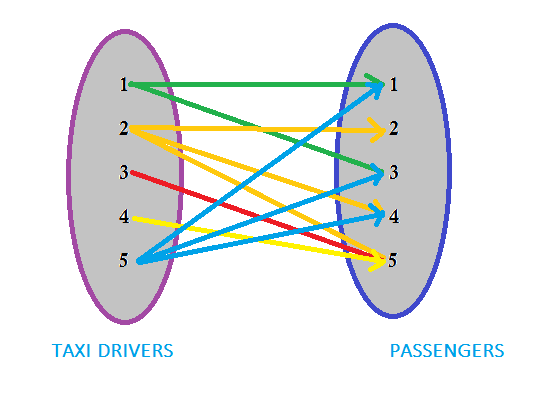# CDVA1608 - Editorial

Tester: Ashutosh Kumar

MEDIUM-HARD

### PREREQUISITES:

Graph, Single Source Shortest Path (Dijkstra Algorithm), Bipartite Matching, MAX FLOW

### PROBLEM:

Given a graph whoes each node represents a building and edges represents roads connceting them. At each building there exists a passenger or a taxi driver, except at the Movie theatre. Each taxi driver will take only one passenger and will drive for limited amount of time. Your task is to compute maximum number of passenger reaching the theatre. Given, each passenger wants to go to the theatre and can approach to any taxi driver.

### QUICK EXPLANATION:

Create a bipartite graph in which first set is for taxi drivers and second one is for passengers. Draw an edge between a taxi driver and a passenger, if and only if, that taxi driver can drop that passenger at the theatre within his driving time. You can use any single source shortest path for this purpose. Now you have a graph, you just need to find maximal matching in it.

### EXPLANATION:

This problem can is divided into two parts: Building graph and computing the Maximum flow.

##Creation of Graph:
We can see that each taxi driver can be approached by all the passengers, but there is a driving time limit for each driver. So, we construct a graph (bipartite) containing two set of nodes, first set for taxi driver and second one for passengers.

### How to draw edges in the graph?

Let’s take a passenger j and driver i. There exist an edge in the graph between them, if and only if, the taxi driver drops the passenger to the theatre, i.e., T* * S* >= (distTaxi*[j] + distTh[j]), where T*, S* and distTaxi*[j] represents driving time, speed, and minimum distance (between i_{th} driver and j_{th} passenger) of the i_{th} driver respectively. distTh[j] represents the minimum distance between j_{th} passenger and Movie theatre.
To compute distTh for all passenger, apply SSSP (single sourse shortest path, [Dijkstra Algorithm]) from the Movie theatre. Also, for distTaxi apply SSSP from each taxi driver.

	for each i as taxi driver:
capacity = S* * T*
for each j as passenger:
totaldist = distTh[j] + distTaxi*[j]
if totaldist <= capacity:
We have an edge from i to j.


##Computing Max Flow

Our build graph looks like the one below.Once our graph is build, you just need to compute how many maximum number of edges that you can use to reach the theatre, considering atmost one outgoing edge can be present from each driver, i.e., finding maximal matching of this graph.
Time limit is a bit strict so use fast algorithm for maximal matching and SSSP.

### OPTIMAL COMPLEXITY:

\mathcal{O}(N*R\log(N+P+1) + N*P)

### AUTHOR’S AND TESTER’S SOLUTIONS:

Author’s solution can be found here.

Tester’s solution will be uploaded soon.